# Catch up on the latest AI articles# Inverse Synthetic Analysis Model GTA Using Both Graph And SMILES Representations

3 main points
✔️ The most popular deep learning models for reverse synthesis tasks are based on sequence-to-sequence (seq2seq) and graph neural networks (GNN), and this paper proposes Graph Truncated Attention (GTA), which combines these models
✔️ In GTA, chemicals are represented as two representations, graph representation, and string representation, and the inverse synthesis is performed by a novel graph-truncated attention method using both representations.
✔️ This model achieves state-of-the-art results on the USPTO-50k benchmark and USPTO-full dataset

written by Seo, S.-W., Song, Y. Y., Yang, J. Y., Bae, S., Lee, H., Shin, J., Hwang, S. J., & Yang, E.
(Submitted on 18 May 2021)
Subjects: Machine Learning (cs.LG)code：The images used in this article are from the paper, the introductory slides, or were created based on them.

## first of all

Retrosynthesis is the task of predicting the set of reactant molecules that will be synthesized into a given product molecule by finding a reverse reaction pathway. It is a particularly important task in organic chemistry because finding a synthetic pathway is as difficult as discovering a new compound. Since the advent of the concept of reverse synthesis, chemists have attempted to use computers for reverse synthetic analysis to find candidate reactants in a fast and efficient manner.

With the recent success of deep learning in various chemical tasks and the availability of large datasets, research has begun to emerge to tackle the inverse synthesis problem in a data-driven manner using deep learning. This deep learning-based approach has the potential to solve tasks without human intervention or prior knowledge or expertise and can be a time and cost-efficient approach. Recent approaches to inverse synthesis using deep learning can be classified as template-based and template-free. Template here refers to a set of rules describing how a reactant is transformed into a product using atom-by-atom mapping information. Naturally, expertise is required to translate templates into models, which is why today's state-of-the-art template-based models show higher performance than template-free models. However, responses that are not included in the extracted templates are rarely predicted by template-based models and therefore lack generalization ability. Another drawback is that it takes a lot of time to experimentally validate a template, such as 15 years for 70,000 templates. Template-free models, which learn directly from data, have the advantage of being able to generalize beyond the extracted templates and of eliminating the problem of template validation. The first template-free model proposed was the seq2seq model, which predicts the string representation of a reactant from the string representation of a given product. Transformers, etc. are used as Seq2seq models. The model is still being improved by incorporating a learning rate schedule, adding latent variables, etc. Recent template-free models using graph representations are called graph-to-graph (G2Gs), and while G2Gs reported state-of-the-art performance on the USPTO-50k data set, it still requires some of the same steps as template-based models, such as requiring chemist-labeled atomic mappings. Unlike these models, the GTA model focused for the first time on the duality between graph and string representations and performed an inverse synthesis analysis without adding any parameters to the Transformer model.

## writing on the surface (e.g. an address on an envelope)

SMILES stands for Simplified molecular-input line-entry system. It is widely used for molecular property prediction, molecular design, and reaction prediction. For example, the reaction to synthesize benzene from furan and ethylene is shown in the figure below.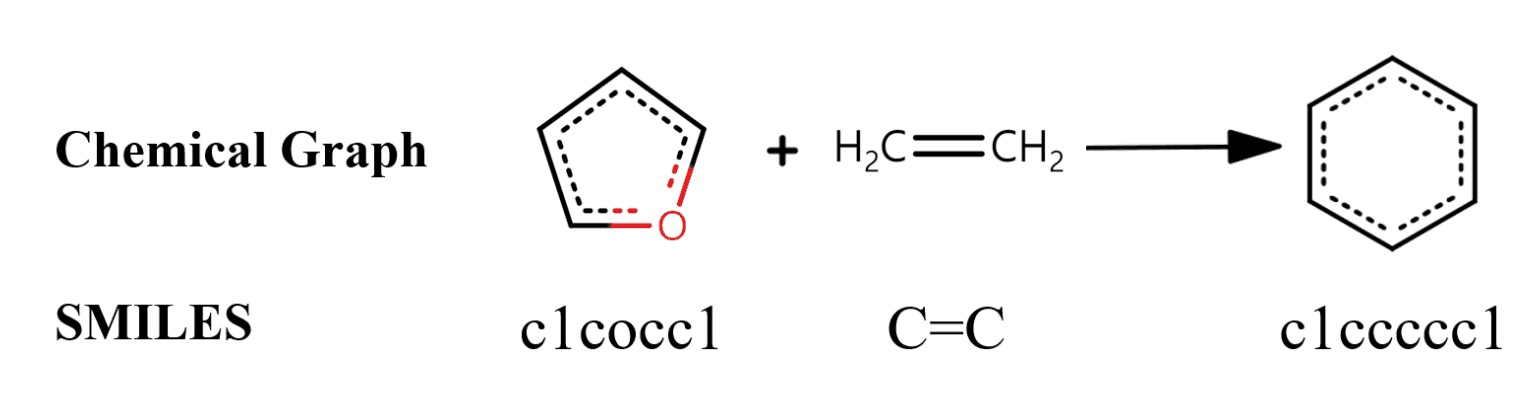## Transformer

The Transformer architecture is currently de facto for solving many natural language processing tasks such as machine translation because it can learn long-range dependencies of tokens by self-attention. In the inverse synthesis task, the Molecular Transformer performed the task of "translating" a set of reactants $\left\{R_1, R_2, \dots\right\}$ into a SMILES representation of the product $P$. The Transformer can be expressed in the following form.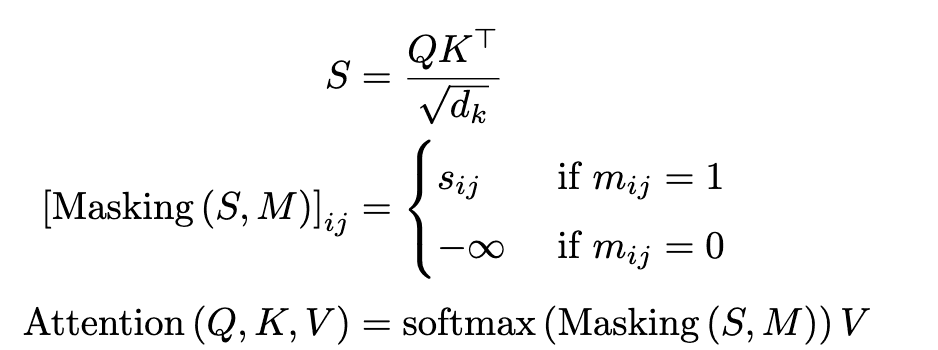However, $Q\in\in\mathbb{R}^{T_m\times d_k}$, $K\in\mathbb{R}^{T_m\times d_k}$, and $V\in\mathbb{R}^{T_m\times d_v}$ are the parameters to be learned, $S=\left(s_{ij}\right right)$ is the score matrix, $M=\left(m_{ij}\right)\in\left\{0, 1\right\}^{T_m\times T_m}$ is the mask matrix. The mask matrix $M$ is customized according to the purpose of each attention module.

## Graph-truncated attention

The goal of GTA is to include information about graph structure in the self-attention and cross-attention layers of Transformer. Inspired by the recent success of using masks in pre-trained language models, we use masks generated from graph information to compute attention mechanisms; in GAT we consider two masks, one in the self-attention layer and one in the cross-attention layer.

## Graph-truncated self-attention (GTA-self)

If the distance between atoms $i$ and $j$ on the molecular graph is $d$ (or if atoms $i$ and $j$ are $d$ hop neighbors), then $m_{ij} = 1$. The $D$ is a hyperparameter and we used $D=1,2,3,4$ in our experiments. If the distance matrix is given as $D=\left(d_{ij}\right)$, the mask corresponding to the $h$-th head is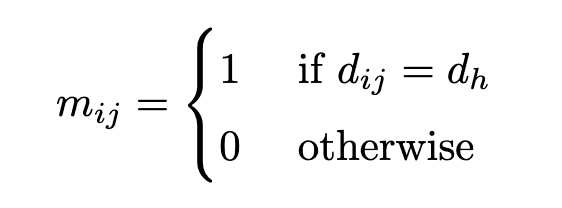The result is the same as the Graph Attention Network (GAT) when all heads have $d_h=1$. It is the same as Graph Attention Network (GAT) when all heads have $d_h=1$. In our experiments, we set $d_h=\left(h\text{ mode }4\right)+1$ using the head index $h$. As an example, a mask with $d_h=1$ for the red atom of benzene is shown on the left in (b) of Figure 2.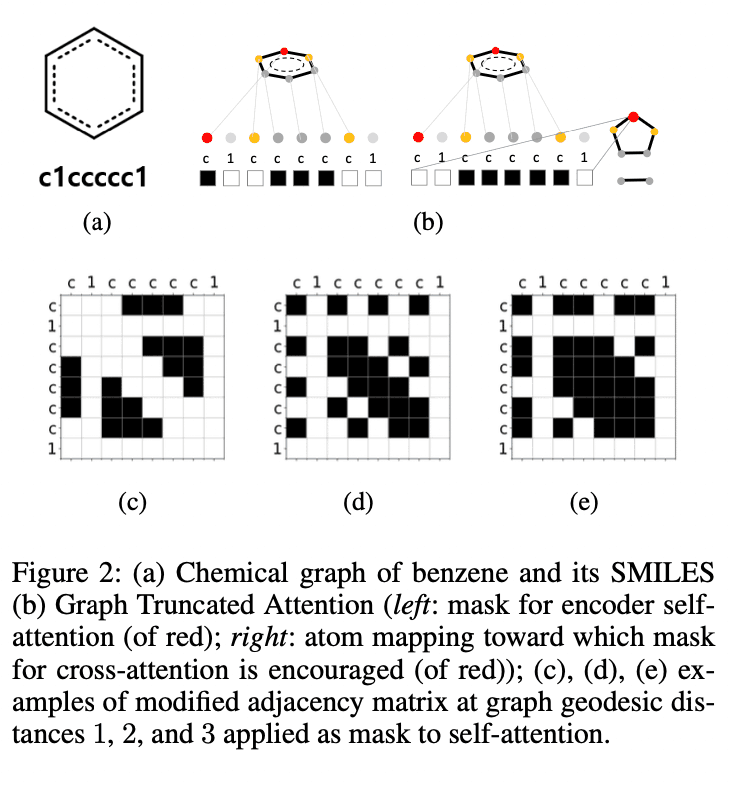## Graph-truncated cross-attention (GTA-cross)

The reaction is not a process of completely breaking down a molecule to make an entirely new product, and the product and reactant molecules usually have a fairly common structure. It is therefore a natural idea to consider a cross-attention layer. However, how to map atoms between products and reactants is not easy, and it is an active research topic in chemistry. In this study, for simplicity, we use the FMCS algorithm implemented in the standard RDkit; based on the (partial) atomic mapping between the product and reactant molecules obtained by the FMCS algorithm, the mask $M=\left(m_{ij}\right)\in\left\{0,1\right \}^{T_R\times T_P}$.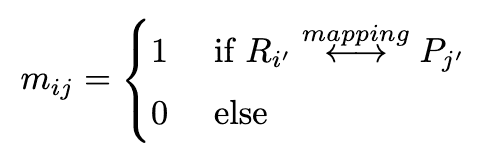We define $R_{i'} where$i'$is the index of the vertex of the graph$G(m)$corresponding to the$i$-th token of$S(m)$,$R_{i'}$is the vertex of the graph$G(R)$and$P_{j'}$is the vertex of the graph$G(P)$. As shown in Figure 3, the cross- The mask for cross-attention is set to 1 if the corresponding atom is matched by atom mapping, and 0 otherwise. The mask generated by the above equation is difficult to find mappings, both because the atomic mappings are incomplete and because it creates incomplete SMILES when generating sequences during inference. Therefore, we used the following loss function to gradually learn the complete atomic mappings. $$\mathcal{L}_{\text {attn }}=\sum\left[\left(M_{\text {cross }}-A_{\text {cross }}\right)^{2} \odot M_{\text {cross }}\right]$$ where$M_{\text {cross }}$is the mask matrix defined above and$A_{\text {cross }}$is the cross-attention matrix. ### loss function Finally, the overall GTA loss is$\mathcal{L}_{\text{total}}=\mathcal{L}_{\text{ce}}+\alpha\mathcal{L}_{\text{attn}}$. Although there seems to be no GTA-self loss term, the effect of GTA-self is implicitly built-in since the self-attention generated by GTA-self contributes to the cross-entropy loss$\mathcal{L}_{\text{ce}}$through the model output. In our experiments$\alpha$is set to$1.0$.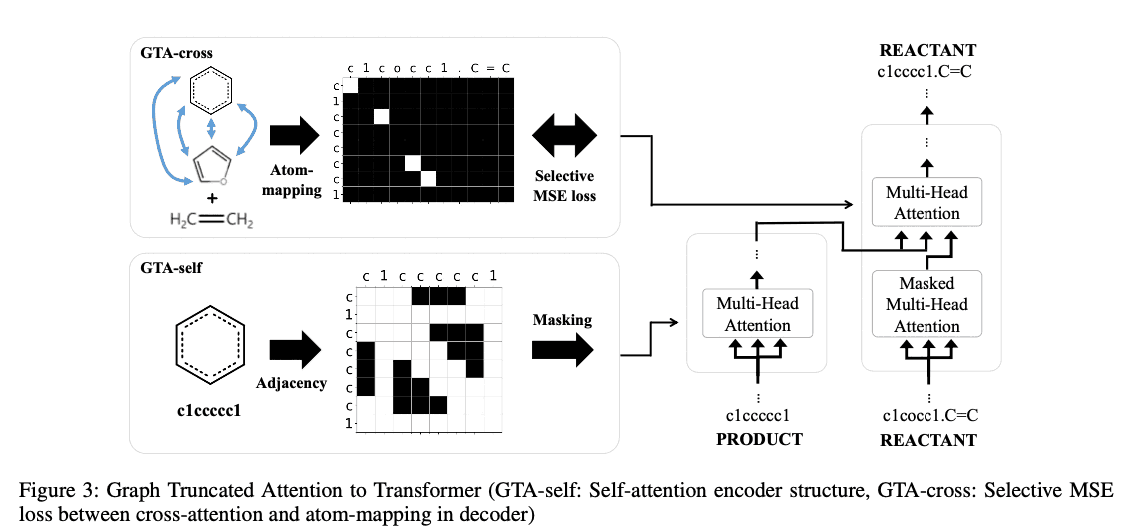## experimental results The experiments were performed using USPTO-50k and USPTO-full, which are open data sets of chemical reactions. Table 1 compares with the existing template-free model and Table 2 compares with the existing template-based model. The values are the Top-k perfect match accuracy (%), and the$\pm\$ symbol is followed by the 95% confidence interval width. The case where the data was expanded by changing the reactant order and starting atoms is written as 2P2R_s.

From the table, GTA exceeded 50 % Tok-1 accuracy for the first time in the template-free model on the UPSTO-50k dataset and outperformed template-based GLNs by 5.7 % and 6.3 % for Top-1 and Top-10 accuracy on the USPTO-full dataset, respectively The top-10 accuracy of the USPTO-full dataset exceeded 50 %.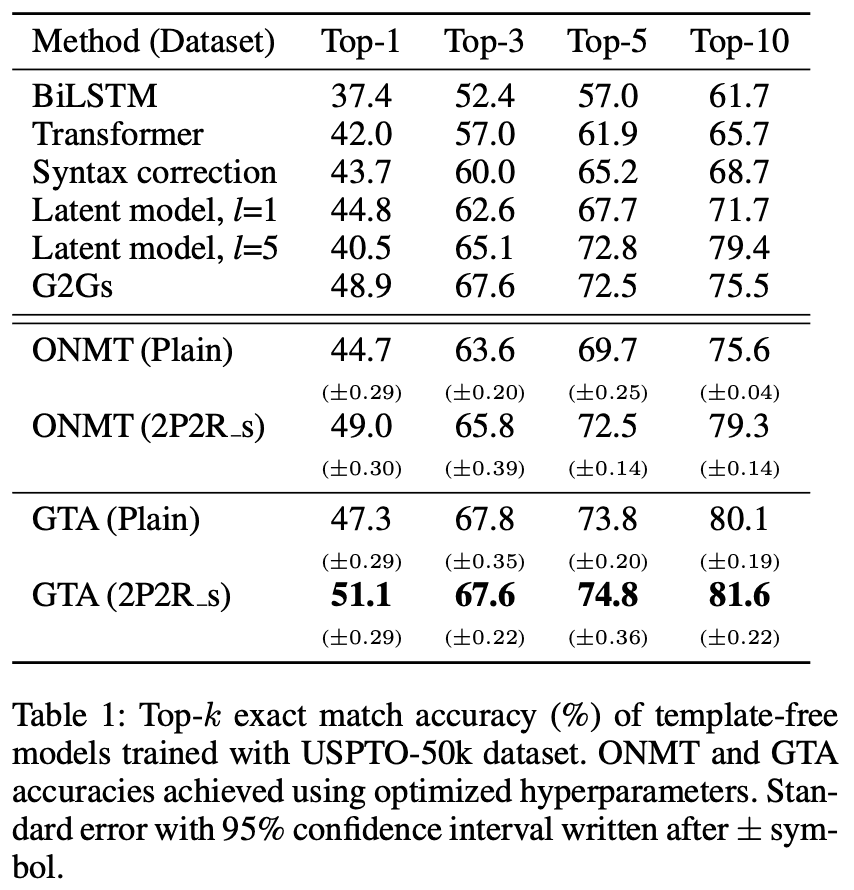## summary

In this study, we proposed a method to solve the inverse synthesis analysis by combining molecular features as both SMILES and graph representations. As a result, we obtained good results compared with both template-free and template-based models.

In this paper, it is demonstrated that the existing Transformer model can be improved by incorporating a mask that takes into account the graph structure. It is veexcitinghat the state-of-the-art results are obtained without any additional parameters since we only incorporate the mask.

If you have any suggestions for improvement of the content of the article,
please contact the AI-SCHOLAR editorial team through the contact form.